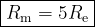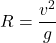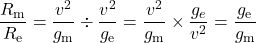Question

Assume that the acceleration due to gravity
on the surface of the moon is 0.2 times the
acceleration due to gravity on the surface
of the earth. If R, is the maximum range of
a projectile on the earth’s surface, what is
the maximum range on the surface of the
moon for the same velocity of projection

1.Explanation:

A projectile has its maximum range when you fire it at an angle of 45°.

The formula becomesIf you are comparing the ranges on the Moon and the Earth,